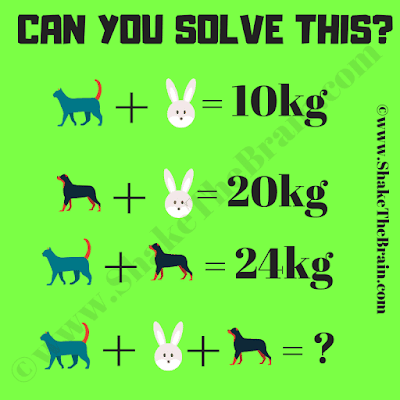Here is the Math Equation Puzzle to test your Mathematical skills. In this Math, equation variables are replaced with animals. Can you find the value of each animal and then solve the last equation?Can you solve this math picture puzzle?

Answer of this "math equation puzzle for teens" can be viewed by clicking on the button. Please do give your best try before looking at the answer.

Unknown said...

27

Unknown said...

27

Unknown said...

These are the wonderful questions that increase our mental abilities